## Forum Posts

Davey Yu
Sep 22, 2022
In ACSL Contest Prep
and also 9/14/22 optional practice because I forgot to put it here So, Recursive Functions. Recursive Functions always look something along the lines of this-> Given f(x) or maybe f(x,y), find a number related to that So, let's say that I'm trying to find f(5) in this sample question. Because 5 is greater than 1, then it falls into the second category. So f(5) = f(4) + f(3) Now we find f(4) and f(3) f(4) = f(3) + f(2) f(3) = f(2) + f(1) f(2) = f(1) + f(0) f(1) = 1 f(0) = 0 And then you fill it up with the info you know f(2) = f(1) + f(0) = 1 + 0 = 1 f(3) = f(2) + f(1) = 1 + 1 = 2 f(4) = f(3) + f(2) = 2 + 1 = 3 f(5) = f(4) + f(3) = 3 + 2 = 5 Therefore, f(5) = 5 Homework: other homework for 9/14 bc i forgor 💀0
1
11
Davey Yu
Sep 08, 2022
In ACSL Contest Prep
Today(yesterday because I made these too late), you learned about Computer Number Systems To Convert from: Hex -> Binary Sample number: 56 5 = 0101 6 = 0110 56H = 1010110 Same process but with 3 digits for octal Binary -> Decimal Sample Number: 110 1 1 0 2^2 + 2^1 = 4+2=6 Anything else that I probably didn't describe, ask it here and I'll respond. - Davey, the TA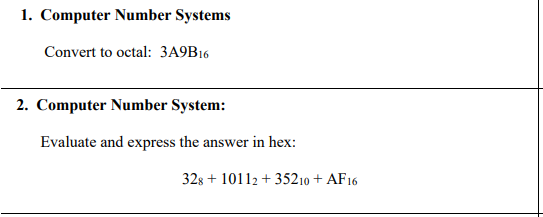0
2
42
Davey Yu
Jul 21, 2022
In ACSL Contest Prep
Digital Electronics Notes: This way of having pictures is super weird so zoom in on the question if you have to.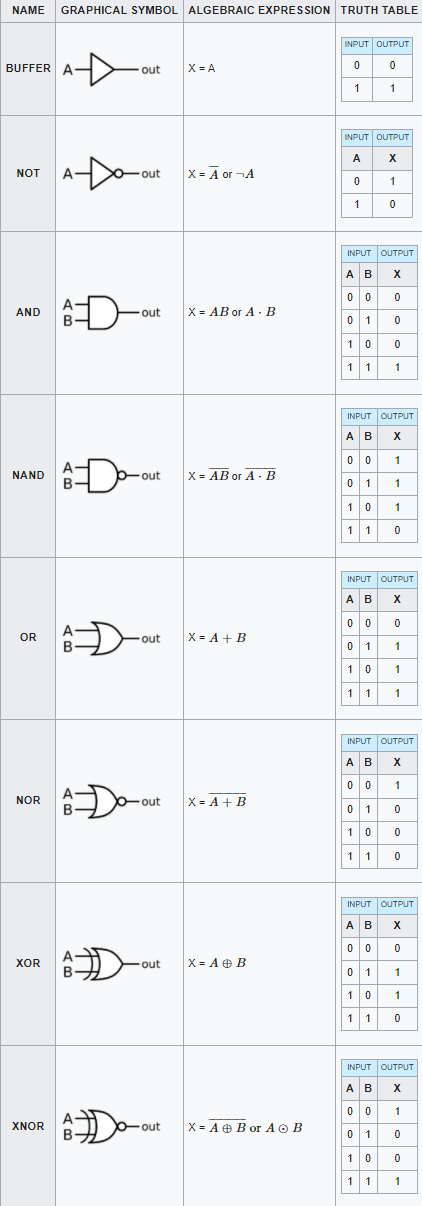0
1
6
Davey Yu
Jul 14, 2022
In ACSL Contest Prep
Stack: They go by the Philosophy: "Last in, First out" A can of chips or times when the way in is the way out. Queue: They go by the Philosophy: "First in, First out" A straight line, where you would wait for a while. Binary Search Tree: A tree that uses the alphabet as its measuring tape. ABCDEFGHIJKLMNOPQRSTUVWXYZ For Example: Coding C O > C so O will go to the right of C D > C so D 1718 INTJR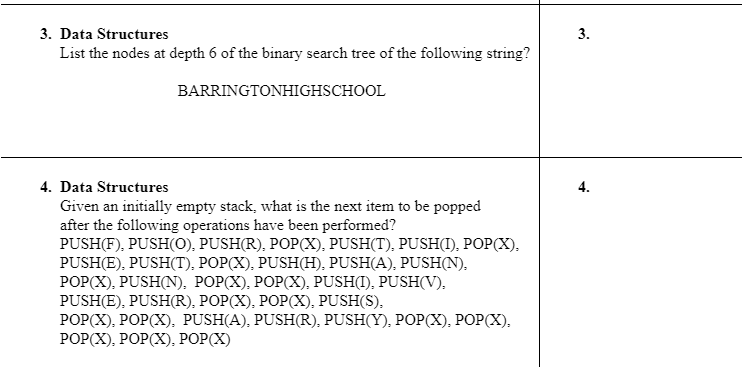0
0
6
Davey Yu
Jun 23, 2022
In ACSL Contest Prep
Class Notes: Shifts - Shift to the right or left Circ - Moves bits from the right to the left and vice versa Order of Operations(I'm giving this to you during this homework but normally you are expected to memorize it) (and trust me, you will need it) XOR < OR < AND < NOT NOT 0 -> 1, 1 ->0 AND Best known as Multiplication 0 AND 1 -> 0 0 AND 0 -> 0 1 AND 1 -> 1 OR Best known as addition 0 OR 1 -> 1 0 OR 0 -> 0 1 OR 1 -> 1 XOR Are they the same? If yeah, 0. If no, 1 0 XOR 0 -> 0 0 XOR 1 -> 1 1 XOR 1 -> 00
2
15
Davey Yu
Jun 09, 2022
In ACSL Contest Prep
Today, we learned about Recursive Functions, functions that loop repeatedly. Hey you, you're finally awake. Comment your answers on this post. If you need any sort of help, I'll answer. 20192020 IN0
6
67
Davey Yu
Mar 18, 2022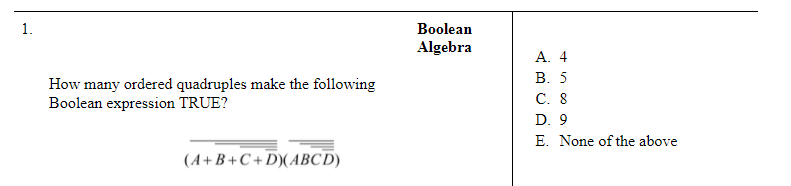0
0
27
Davey Yu
Mar 11, 20220
2
15
Davey Yu
Mar 03, 2022
In ACSL Contest Prep
Today, we review our first 3 units. HW(All of this is from 1617 All-Star Junior.)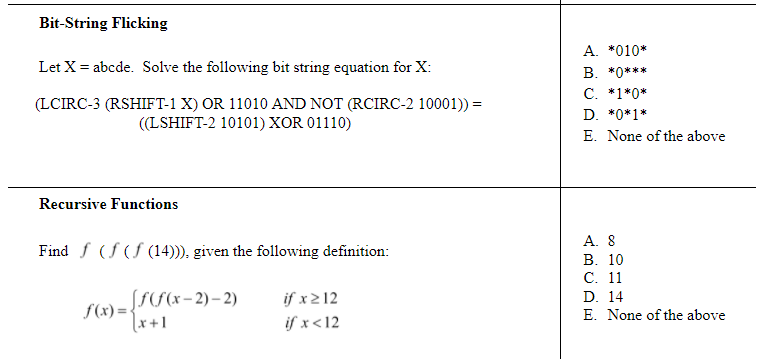0
4
29
Davey Yu
Feb 17, 2022
In ACSL Contest Prep
Today, we learn how to identify and solve questions in Assembly Language. 17-18 S4 1920 I40
2
15
Davey Yu
Feb 03, 2022
In ACSL Contest Prep
Today, we talk about the graphs and the paths and cycles between them. From 2017-2018 Junior Contest 4 2. What are the different number of unique cycles in this graph? 3. Draw the following graph using the matrix below0
4
38
Davey Yu
Jan 13, 2022
In ACSL Contest Prep
Homework: From Senior Contest 3 2019-2020 2. From Senior Contest 3 2017-2018 3. From AS Senior Level 2017-20180
4
36
Davey Yu
Jan 06, 2022
In ACSL Contest Prep
Since Tyler couldn't make it today, I will be explaining our new topic, Data Structures. There are 4 types of Data Structures: Stacks Queues Binary Search Trees Priority Queues Let's take this example: In Stacks and Queues, You'll see the methods PUSH and POP. PUSH inserts an item into the stack/queue. POP has different effects based on whether it's a Stack or a Queue. When using POP, Stacks uses the rule "First in, Last out." This signifies that POP would take out the most recent item inserted. For example, the first POP in this stack would remove R from the stack. On the other hand, Queues use the rule "First in, First out." This signifies that POP would take out the thing in the first "slot." In this case, the first POP in the above queue would remove F, unlike the R it would remove if it was a Stack. ------------------------------------------------------------------------------------------------------------------------- i was never good at this part of this it might suck A tree has these features. The "root" is the first node, its "children" is the following few nodes. Take the Example, ACSL A is the top and Z is the bottom when it comes to higher and lower A is our first letter, so it starts as the root of our tree. C is "less" or "lower" than A alphabetically, so it goes to the right of the parent. S is "lower" than C and A on the alphabet, so it goes to the right of C. L is "higher" than S on the alphabet, but "lower" than A and C, so it goes to the left of S. And that is our Binary Search Tree of ACSL. I'm going to make a funny joke, so we are going to make a Binary Search tree of "Amogus" Our first step is to set A as our parent node. Then we continue with the same logic as always. M is "lower" on the alphabet than A, so it goes to the right of A. O is "lower" than both A and M, so it goes to the right of M. G is "lower" than A, but "higher" than M, so it goes to the left of M. The Binary Search tree should look like this when you're done: I didn't mention everything, so if you're confused about something, let me know in the comments. -------------------------------------------------------------------------------------------------------------------- Priority Queues I'm actually not too good with this, so, I suggest you look it up or make the slides that I hope Tyler made. Now for the actual HW part: 1. 2 & 3.0
3
26
Davey Yu
Dec 16, 2021
In ACSL Contest Prep
We learned about Boolean Algebra and the functions of AND, OR, NOT, XOR, XNOR, and NAND. From Intermediate Contest 3 2017-2018 From Senior Contest 3 2019-2020 is XOR 5. Make a Truth Table to the best of your ability of the following (It's ok if you don't understand/finish this question, this question is time consuming and hard too)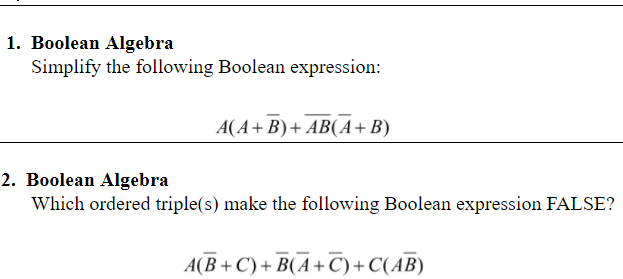0
5
35
Davey Yu
Dec 02, 2021
In ACSL Contest Prep
Today, we learned the functions of LISP and how to make our own functions using DEF or DEFUN. Make your own function. From Intermediate Contest 2 2017 - 2018 3. From Intermediate Contest 2 2015 - 2016 4. From Senior Contest 2013-2014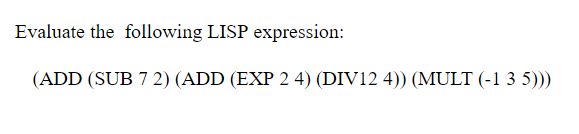0
5
29
Davey Yu
Nov 18, 2021
In ACSL Contest Prep
The operations such as OR, AND, NOR, and XOR are not the only type of Bitstring Flicking. Stuff like LShift and RCirc also exist too. 1. RSHIFT-1(LSHIFT-1(RSHIFT-1(LSHIFT-1(RSHIFT-1(LSHIFT-1(11011))))) 2. LCIRC-3(10010 OR NOT(01101)) AND RSHIFT-2(NOT(RCIRC-1(00111 OR NOT(10111))) 3 & 4: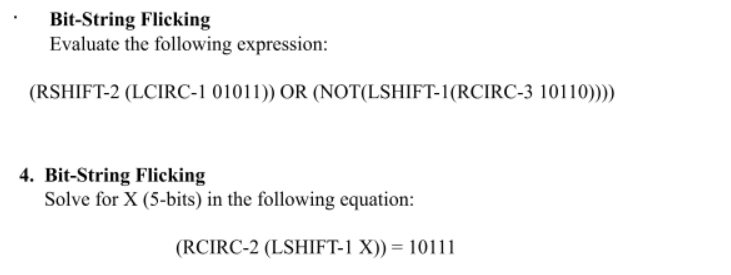0
4
30
Davey Yu
Nov 12, 2021
In ACSL Contest Prep
1 & 2. From 2017-2018 Junior Contest 2 3 & 4. From 2016-2017 Junior Contest 20
5
27
Davey Yu
Oct 28, 2021
In ACSL Contest Prep
1. All Numbers are single digits From Intermediate Contest 2 2016-2017 2. From Intermediate Contest 2 2017-2018 3. From Intermediate Contest 2 2015-2016 4. From Intermediate Contest 2 2015-2016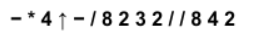1
5
32
Davey Yu
Oct 14, 2021
In ACSL Contest Prep
1. What is outputted when this program is run? a = 1: b = 2: c = 3: d = 4: e = 4: f = 6 if (d / b) < (f / a) then d = d / b a = f ↑ b / c ↑ (d / b) if (a <= f) && (b > e) then a = f else b = e if abs(c - f) != int(f / c) then c = f / c else f = f / c if (a = = b) | | (c = = d) then a = a + b c = c + d output (b * c) * (f + d) / a / 2 * d - c + e ↑ (b - 2 * d) From Intermediate Contest 1 2017-2018 2. From Junior Contest 2 2016-2017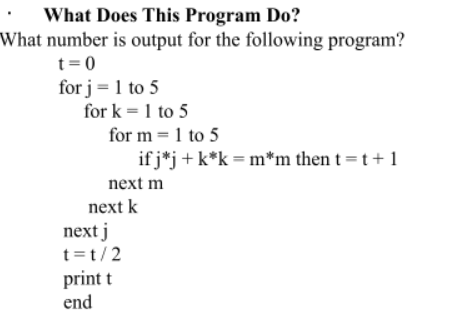0
5
34
Davey Yu
Oct 07, 2021
In ACSL Contest Prep
How would you be able to use a 2D array in a real-world situation? What does len(string) do? How would you be able to print an array to make a string, assuming the array was a char array? Beginning with an initially empty array A, what is the positive difference between the maximum and minimum non-zero values in the array after the program is run? for n = 1 to 4 for p = 1 to 4 a(n,p) = n + p + n*p next p next n for n = 1 to 4 for p = 1 to 4 if (a(n,p) / 2 = int(a(n,p) / 2)) || (a(n,p) / 3 = int(a(n,p) / 3)) || (a(n,p) / 5 = int(a(n,p) / 5)) then a(n,p) = 0 next p next n end From Junior Contest 3 2017-2018 5. From Junior Contest 1 2015-20160
2
64
D

Forum Moderator
More actions